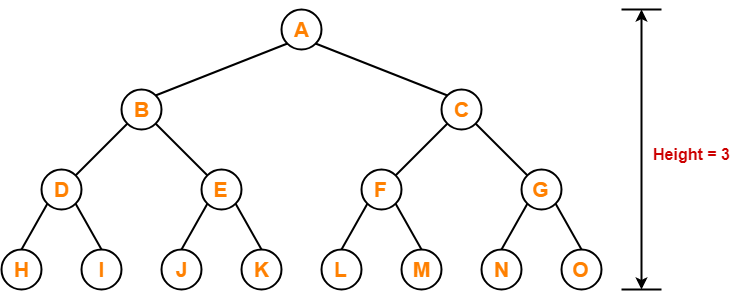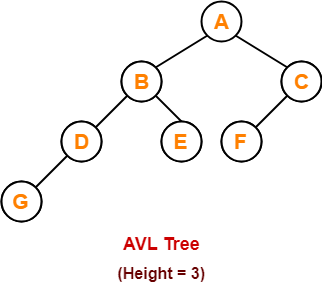# AVL Tree Properties | Problems on AVL Tree

## AVL Tree-

Before you go through this article, make sure that you have gone through the previous article on AVL Trees.

We have discussed-

• AVL trees are self-balancing binary search trees.
• In AVL trees, balancing factor of each node is either 0 or 1 or -1.

## AVL Tree Properties-

Important properties of AVL tree are-

## Property-01:

 Maximum possible number of nodes in AVL tree of height H = 2H+1 – 1

### Example-

Maximum possible number of nodes in AVL tree of height-3

= 23+1 – 1

= 16 – 1

= 15

Thus, in AVL tree of height-3, maximum number of nodes that can be inserted = 15.We can not insert more number of nodes in this AVL tree.

## Property-02:

 Minimum number of nodes in AVL Tree of height H is given by a recursive relation- N(H) = N(H-1) + N(H-2) + 1

Base conditions for this recursive relation are-

• N(0) = 1
• N(1) = 2

### Example-

Minimum possible number of nodes in AVL tree of height-3 = 7(For explanation, Refer problem-01)

## Property-03:

 Minimum possible height of AVL Tree using N nodes = ⌊log2N⌋

### Example-

Minimum possible height of AVL Tree using 8 nodes

= ⌊log28⌋

= ⌊log223

= ⌊3log22⌋

= ⌊3⌋

= 3

## Property-04:

 Maximum height of AVL Tree using N nodes is calculated using recursive relation- N(H) = N(H-1) + N(H-2) + 1

Base conditions for this recursive relation are-

• N(0) = 1
• N(1) = 2

## NOTE-

• If there are n nodes in AVL Tree, its maximum height can not exceed 1.44log2n.
• In other words, Worst case height of AVL Tree with n nodes = 1.44log2n.

## Problem-01:

Find the minimum number of nodes required to construct AVL Tree of height = 3.

## Solution-

We know, minimum number of nodes in AVL tree of height H is given by a recursive relation-

 N(H) = N(H-1) + N(H-2) + 1

where N(0) = 1 and N(1) = 2

### Step-01:

Substituting H = 3 in the recursive relation, we get-

N(3) = N(3-1) + N(3-2) + 1

N(3) = N(2) + N(1) + 1

N(3) = N(2) + 2 + 1 (Using base condition)

N(3) = N(2) + 3 …………(1)

To solve this recursive relation, we need the value of N(2).

### Step-02:

Substituting H = 2 in the recursive relation, we get-

N(2) = N(2-1) + N(2-2) + 1

N(2) = N(1) + N(0) + 1

N(2) = 2 + 1 + 1 (Using base conditions)

∴ N(2) = 4 …………(2)

### Step-03:

Using (2) in (1), we get-

N(3) = 4 + 3

∴ N(3) = 7

Thus, minimum number of nodes required to construct AVL tree of height-3 = 7.

## Problem-02:

Find the minimum number of nodes required to construct AVL Tree of height = 4.

## Solution-

We know, minimum number of nodes in AVL tree of height H is given by a recursive relation-

 N(H) = N(H-1) + N(H-2) + 1

where N(0) = 1 and N(1) = 2

### Step-01:

Substituting H = 4 in the recursive relation, we get-

N(4) = N(4-1) + N(4-2) + 1

N(4) = N(3) + N(2) + 1 …………(1)

To solve this recursive relation, we need the value of N(2) and N(3).

### Step-02:

Substituting H = 2 in the recursive relation, we get-

N(2) = N(2-1) + N(2-2) + 1

N(2) = N(1) + N(0) + 1

N(2) = 2 + 1 + 1 (Using base conditions)

∴ N(2) = 4 …………(2)

### Step-03:

Substituting H = 3 in the recursive relation, we get-

N(3) = N(3-1) + N(3-2) + 1

N(3) = N(2) + N(1) + 1

N(3) = 4 + 2 + 1 (Using (2) and base condition)

∴ N(3) = 7 …………(3)

### Step-04:

Using (2) and (3) in (1), we get-

N(4) = 7 + 4 + 1

∴ N(4) = 12

Thus, minimum number of nodes required to construct AVL tree of height-4 = 12.

## Problem-03:

What is the maximum height of any AVL tree with 10 nodes?

## Solution-

For calculating the maximum height of AVL tree with n nodes, we use a recursive relation-

 N(H) = N(H-1) + N(H-2) + 1

### Step-01:

Substituting H = 2 in the recursive relation, we get-

N(2) = N(2-1) + N(2-2) + 1

N(2) = N(1) + N(0) + 1

N(2) = 2 + 1 + 1 (Using base conditions)

∴ N(2) = 4 …………(1)

So, minimum number of nodes required to construct AVL tree of height-2 = 4.

### Step-02:

Substituting H = 3 in the recursive relation, we get-

N(3) = N(3-1) + N(3-2) + 1

N(3) = N(2) + N(1) + 1

N(3) = 4 + 2 + 1 (Using (1) and base condition)

∴ N(3) = 7 …………(2)

So, minimum number of nodes required to construct AVL tree of height-3 = 7.

### Step-03:

Substituting H = 4 in the recursive relation, we get-

N(4) = N(4-1) + N(4-2) + 1

N(4) = N(3) + N(2) + 1

N(4) = 7 + 4 + 1 (Using (1) and (2))

∴ N(4) = 12

So, minimum number of nodes required to construct AVL tree of height-4 = 12.

But given number of nodes = 10 which is less than 12.

Thus, maximum height of AVL tree that can be obtained using 10 nodes = 3.

## Problem-04:

What is the maximum height of any AVL tree with 77 nodes?

## Solution-

For calculating the maximum height of AVL tree with n nodes, we use a recursive relation-

 N(H) = N(H-1) + N(H-2) + 1

### Step-01:

Substituting H = 2 in the recursive relation, we get-

N(2) = N(2-1) + N(2-2) + 1

N(2) = N(1) + N(0) + 1

N(2) = 2 + 1 + 1 (Using base conditions)

∴ N(2) = 4 …………(1)

So, minimum number of nodes required to construct AVL tree of height-2 = 4.

### Step-02:

Substituting H = 3 in the recursive relation, we get-

N(3) = N(3-1) + N(3-2) + 1

N(3) = N(2) + N(1) + 1

N(3) = 4 + 2 + 1 (Using (1) and base condition)

∴ N(3) = 7 …………(2)

So, minimum number of nodes required to construct AVL tree of height-3 = 7.

### Step-03:

Substituting H = 4 in the recursive relation, we get-

N(4) = N(4-1) + N(4-2) + 1

N(4) = N(3) + N(2) + 1

N(4) = 7 + 4 + 1 (Using (1) and (2))

∴ N(4) = 12 …………(3)

So, minimum number of nodes required to construct AVL tree of height-4 = 12.

### Step-04:

Substituting H = 5 in the recursive relation, we get-

N(5) = N(5-1) + N(5-2) + 1

N(5) = N(4) + N(3) + 1

N(5) = 12 + 7 + 1 (Using (2) and (3))

∴ N(5) = 20 …………(4)

So, minimum number of nodes required to construct AVL tree of height-5 = 20.

### Step-05:

Substituting H = 6 in the recursive relation, we get-

N(6) = N(6-1) + N(6-2) + 1

N(6) = N(5) + N(4) + 1

N(6) = 20 + 12 + 1 (Using (3) and (4))

∴ N(6) = 33 …………(5)

So, minimum number of nodes required to construct AVL tree of height-6 = 33.

### Step-06:

Substituting H = 7 in the recursive relation, we get-

N(7) = N(7-1) + N(7-2) + 1

N(7) = N(6) + N(5) + 1

N(7) = 33 + 20 + 1 (Using (4) and (5))

∴ N(7) = 54 …………(6)

So, minimum number of nodes required to construct AVL tree of height-7 = 54.

### Step-07:

Substituting H = 8 in the recursive relation, we get-

N(8) = N(8-1) + N(8-2) + 1

N(8) = N(7) + N(6) + 1

N(8) = 54 + 33 + 1 (Using (5) and (6))

∴ N(8) = 88 …………(6)

So, minimum number of nodes required to construct AVL tree of height-8 = 88.

But given number of nodes = 77 which is less than 88.

Thus, maximum height of AVL tree that can be obtained using 77 nodes = 7.

Next Article- Insertion in AVL Tree

Get more notes and other study material of Data Structures.

Watch video lectures by visiting our YouTube channel LearnVidFun.

SummaryArticle Name
AVL Tree Properties | Problems on AVL Tree
Description
AVL tree is a self balancing binary search tree data structure. AVL Tree Properties are given. If height of AVL tree = H then, minimum number of nodes in AVL tree is given by a recursive relation N(H) = N(H-1) + N(H-2) + 1.
Author
Publisher Name
Gate Vidyalay
Publisher Logo